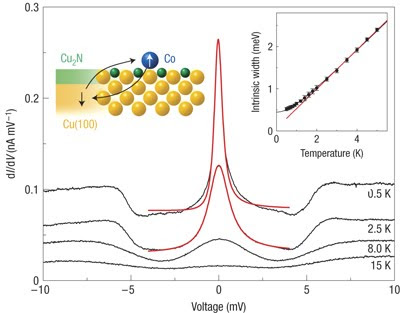## Wednesday, April 14, 2010

### Towards understanding the Kondo problem

This week's Quantum many-body theory reading group is reviewing some basics of scattering theory since that is so key to understanding some aspects of the Anderson single impurity model (Ch. 6 in Phillips) and the Kondo effect (Ch. 7).

Here are a few of the key ideas.
A nice treatment of some of the issues is also in Chapter 2 of The Kondo Problem to Heavy Fermions by A.C. Hewson.

Elastic scattering theory can be formulated in terms of phase shifts in different angular moment channels. The phase shift can be related to the T-matrix (equation 6.38).
Strongest scattering (unitary scattering) corresponds to a phase shift of pi/2.

When the scattering is strong one needs to calculate the T-matrix from the self-consistent equation
T = V + VGT (6.33)
where G is the full electron Greens function which is given by the Dyson equation
G=G0 + G0VG (6.32)
Both of these equations have nice diagrammatic interpretations.This figure is taken from this paper.